# SVC Sort Results VI

## Sound and Vibration Measurement Help

Edition Date: March 2018
Part Number: 372416L-01
View Product InfoLabVIEW 2018 Sound and Vibration Toolkit HelpLabVIEW 2019 Sound and Vibration Toolkit Help

Owning Palette: Spectrum Extended Measurements VIs

Requires: Sound and Vibration Toolkit

Sorts an array of peaks according to the sort by and sort order parameters. Use this VI to sort peaks that the SVFA Spectrum Peak Search, SVT Spurious Free Dynamic Range, and SVT THD and Harmonic Components VIs return. Wire data to the peaks in input to determine the polymorphic instance to use or manually select the instance.

You can use this VI to sort peaks in both the frequency and order domains. You can sort peaks by frequency/order or by amplitude and in ascending or descending order.

Use the pull-down menu to select an instance of this VI.

 Select an instance SVC Sort Results (Peaks)SVC Sort Results (XY)

## SVC Sort Results (Peaks)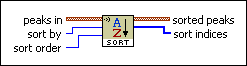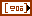peaks in specifies the peaks this VI sorts.X specifies the X, or frequency/order, component of a peak.Y specifies the Y, or amplitude, component of a peak.sort by specifies whether this VI sorts peaks according to frequency/order or amplitude values.

 0 X—Specifies this VI sorts peaks by frequency/order values. 1 Y (default)—Specifies this VI sorts peaks by amplitude values.sort order specifies whether this VI sorts peaks in ascending or descending order.

 0 Ascending—Sorts the peaks from lowest to highest. 1 Descending (default)—Sorts the peaks from highest to lowest.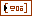sorted peaks returns the sorted peaks. The sorted peaks are arranged in an array consisting of the frequency and the amplitude of each sorted peak.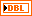X returns the X, or frequency/order, component of a peak.Y returns the Y, or amplitude, component of a peak.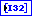sort indices returns the indices of the peaks in array as ordered in the sorted peaks array. You can use the sort indices output to keep other peaks arrays consistent with the sorted peaks array.

Refer to the Details section of this topic for information about using this parameter.

## SVC Sort Results (XY)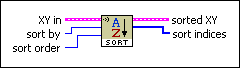XY in specifies XY pairs of frequency/order and amplitude values this VI sorts. The X array contains frequency/order values, and the Y array contains the corresponding amplitude values.X specifies an array of frequency/order values this VI sorts. Each element is part of an XY pair. The X array contains the X, or frequency/order, values that correspond with each element of the Y array.Y specifies an array of amplitude values this VI sorts. Each element is part of an XY pair. The Y array contains the Y, or amplitude, values that correspond with each element of the X array.sort by specifies whether this VI sorts peaks according to frequency/order or amplitude values.

You can choose from the following options:

 0 X (default)—Specifies this VI sorts peaks by frequency/order values. 1 Y—Specifies this VI sorts peaks by amplitude values.sort order specifies whether this VI sorts peaks in ascending or descending order.

You can choose from the following options:

 0 Ascending (default)—Sorts the peaks from lowest to highest. 1 Descending—Sorts the peaks from highest to lowest.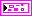sorted XY returns the sorted XY pairs of frequency/order and amplitude values. The X array contains frequency/order values, and the Y array contains the corresponding amplitude values.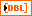X returns the sorted array of frequencies/orders. Each element is part of an XY pair. The X array contains the X, or frequency/order, values that correspond with each element of the Y array.Y returns the sorted array of amplitudes. Each element is part of an XY pair. The Y array contains the Y, or amplitude, values that correspond with each element of the X array.sort indices returns the indices of the XY in cluster as ordered in the sorted XY cluster. You can use the sort indices output to keep other XY clusters consistent with the sorted XY cluster.

Refer to the Details section of this topic for information about using this parameter.

## SVC Sort Results Details

The following figure shows how you can use the sort indices output to sort another array based on the conditions you specify for the SVC Sort Results VI.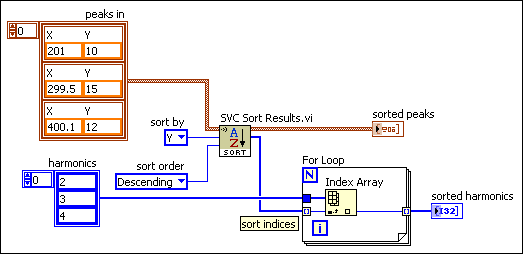In the previous figure, the nth element of the harmonics array corresponds to the nth element of the peaks in array. For example, the peak (201, 10), which is the first element of the peaks in array, corresponds to the value 2, which is the first element of the harmonics array. In this situation, the value 2 represents the second harmonic.

The VI in the previous figure sorts the peaks in array by descending Y values. After this sort operation completes, the elements of the harmonics array no longer correspond with the elements of the sorted peaks array. For example, the peak (201, 10) now is the third array element. However, the third element of the harmonics array is 4, not 2.

To address this issue, the VI uses the sort indices output to sort the harmonics array according to the order of elements of the sorted peaks array. The value of the sort indices output is [1 2 0]. After this sort operation completes, the elements of the sorted harmonics array correspond to the elements of the sorted peaks array.

The value of the sorted harmonics array is [3 4 2]. Notice that the third element of this array is 2. This position corresponds to the (201, 10) peak, which is the third element of the sorted peaks array.

The following figure shows another example of using the sort indices output.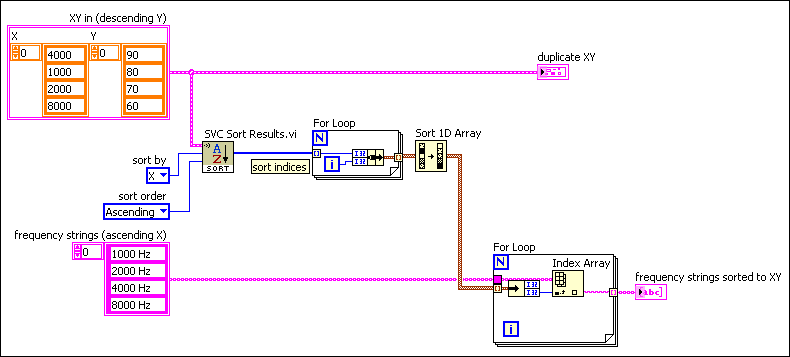The goal of the VI in the previous figure is to sort the frequency strings (ascending X) array to match these same values as sorted in the XY in (descending Y) array. However, notice that these two arrays are sorted in advance by using different criteria. This VI executes the following steps:

1. Sorts the XY in (descending Y) array by ascending X values. These sort criteria match the criteria by which the frequency strings (ascending X) array already is sorted.
2. Uses the sort indices output to determine the order of elements by which the XY in (descending Y) array now is sorted. This output has the value [1 2 0 3].
3. Uses a For Loop to bundle each element of the sort indices array into a cluster. The first element of this cluster is the value from the sort indices array. The second element of this cluster is the loop iteration.

This cluster has the value [1 2 0 3; 0 1 2 3].
4. Sorts the cluster by using the Sort 1D Array function. This function always sorts by ascending values of the first cluster element. This sorted cluster has the value [0 1 2 3; 2 0 1 3].
5. Sorts the frequency strings (ascending X) array according to the second element of this cluster, or [2 0 1 3].

After this sort operation completes, the frequency strings sorted to XY array has the following value: [4000 1000 2000 8000]. Notice these values are elements 2, 0, 1, and 3 from the original array. This order matches the order in which these values exist in the XY in (descending Y) array.